Function Repository Resource:

# ExtractPlotImageData

Extract data from a plot image

Contributed by: Hamza E. Alsamraee
 ResourceFunction["ExtractPlotImageData"][img] converts the plot image img to a normalized set of points. ResourceFunction["ExtractPlotImageData"][img,{xscale,yscale}] scales the results by xscale and yscale.

## Details and Options

The default xscale is based on the image's number of horizontal pixels and the default yscale is 1.
ResourceFunction["ExtractPlotImageData"] takes the mean pixel height of pixels in the same horizontal position.
ResourceFunction["ExtractPlotImageData"] works on a graph of any color on a plain background.
ResourceFunction["ExtractPlotImageData"] is intended to work on images of plots of single-valued functions.

## Examples

### Basic Examples (3)

Extract data from a plot image:

 In:=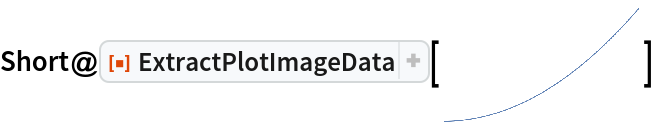Out=An x-Ray diffraction (XRD) pattern of low-density polyethylene (LDPE) converted to a list of points:

 In:=Out=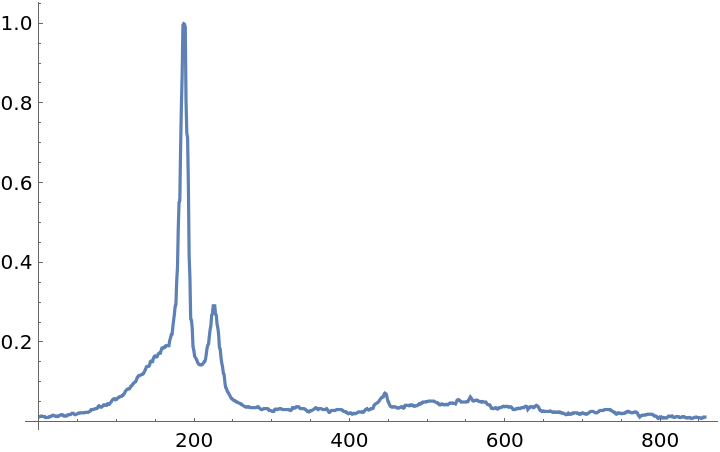Create a Sin plot:

 In:=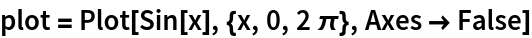Out=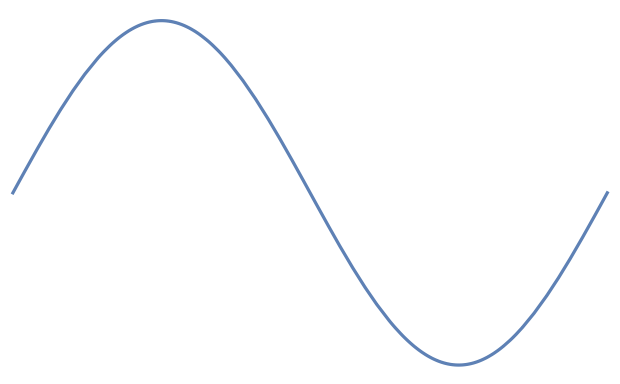Convert the plot into a list of points such that the points are rescaled:

 In:=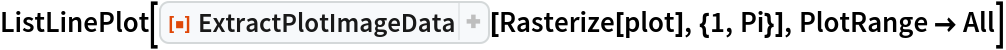Out=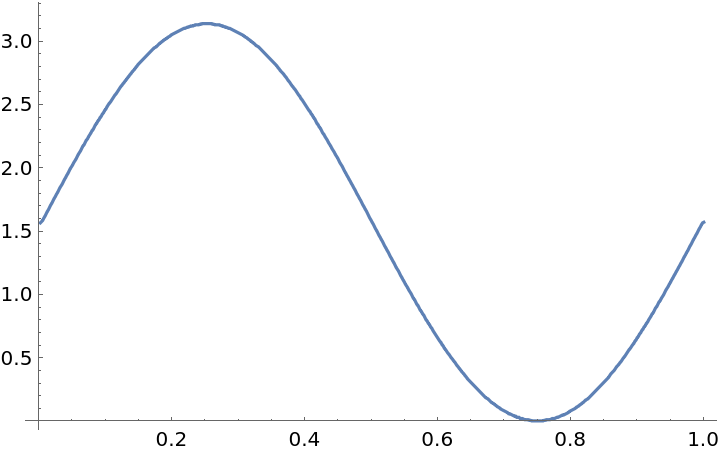### Scope (2)

You can use ExtractPlotImageData on data consisting of points:

 In:=Out=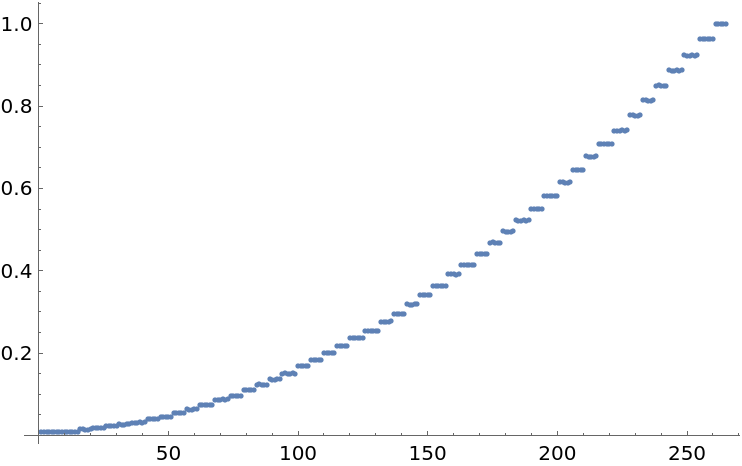The x- and y-scales can be arbitrarily manipulated. Take for example this UV-Vis spectroscopy graph of ZnO nanoparticles:

 In:=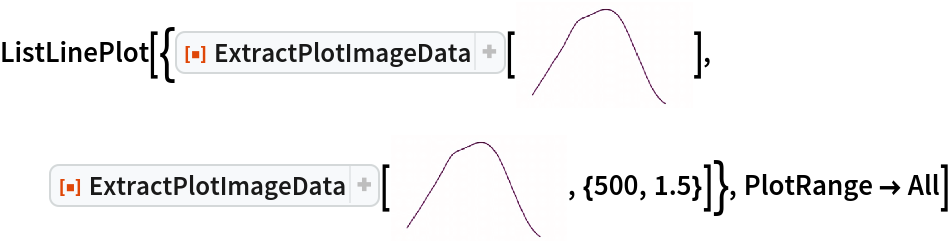Out=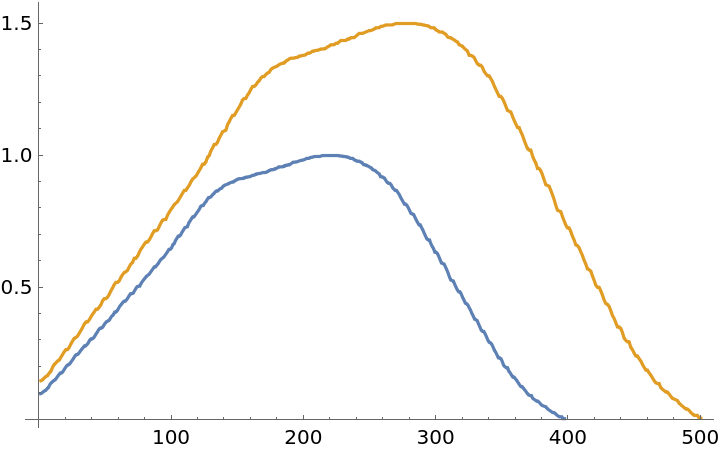### Applications (2)

The extracted data can be interpolated:

 In:=Out=In:=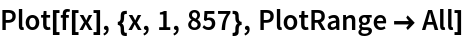Out=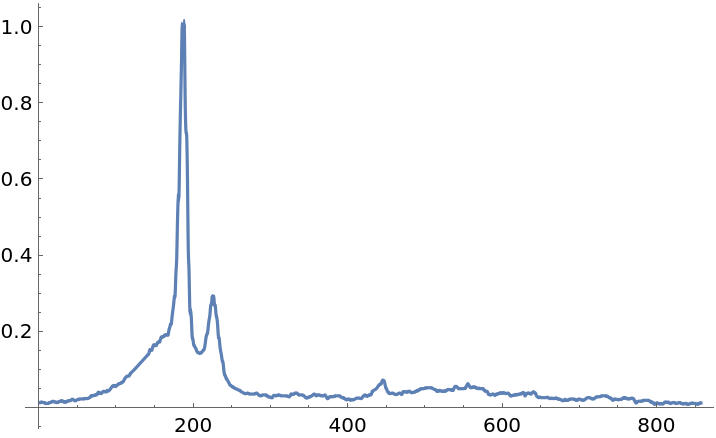On sets of points, FindFormula can be used to detect what relationship is found in the data:

 In:=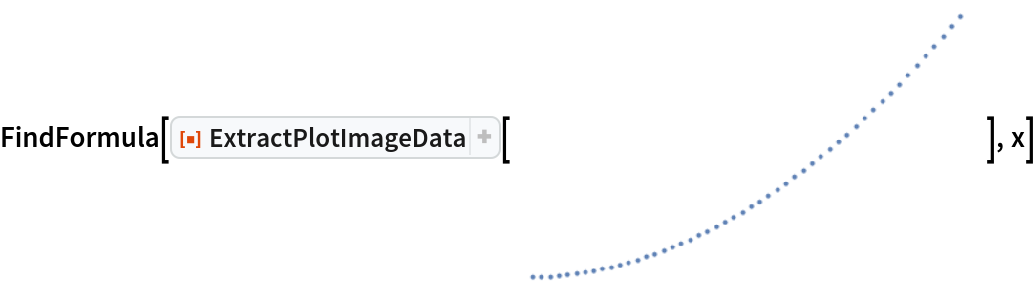Out=### Properties and Relations (1)

For smoother data, a GaussianFilter can be applied:

 In:=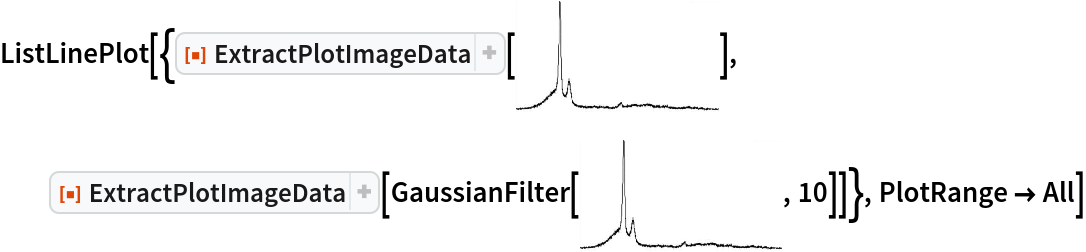Out=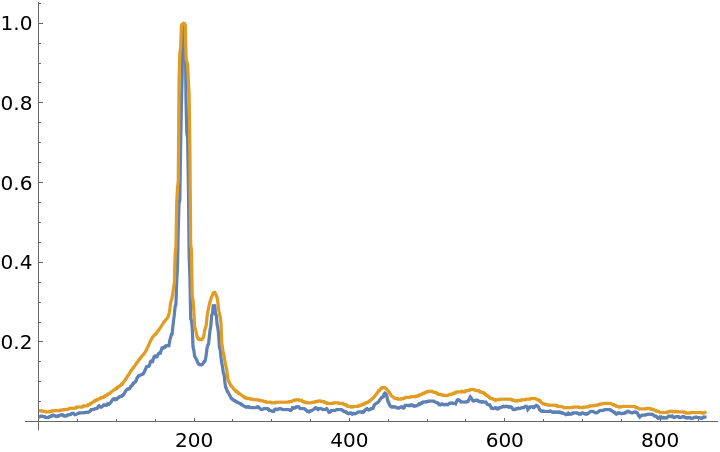### Possible Issues (1)

Content in the image other than the curve is treated as data. Here, the "(a)" distorts the average pixel height:

 In:=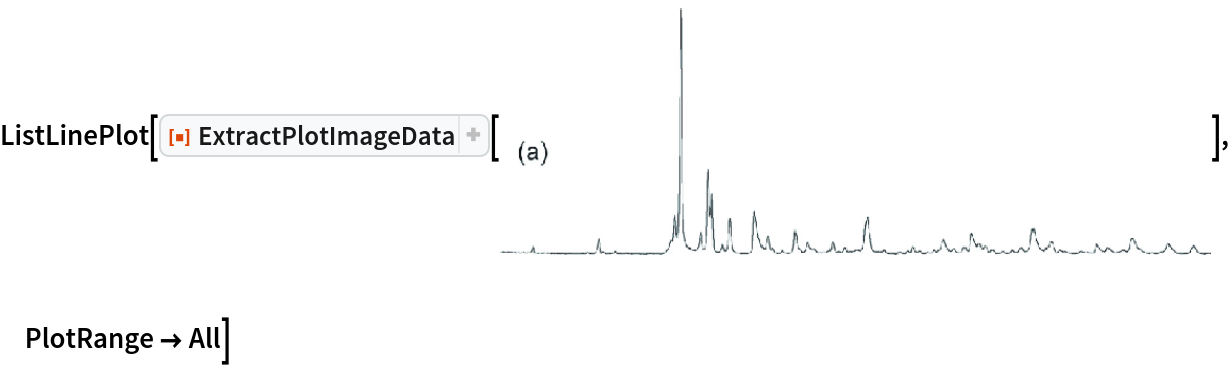Out=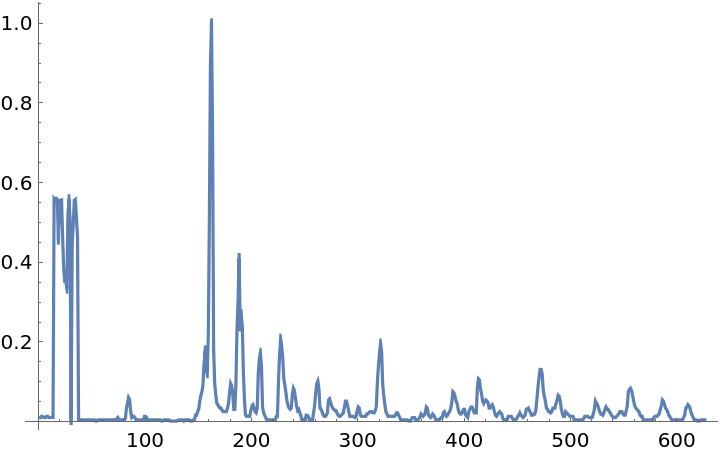## Publisher

Hamza E. Alsamraee

## Version History

• 1.0.0 – 14 July 2020